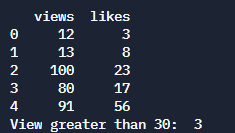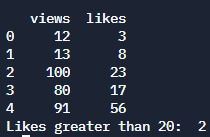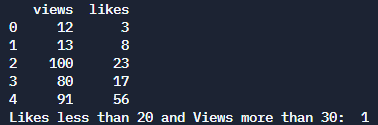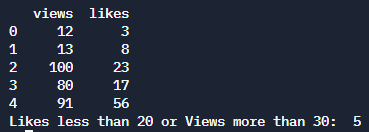# How to Perform a COUNTIF Function in Python?

• Last Updated : 28 Nov, 2021

In this article, we will discuss how to perform a COUNTIF function in Python.

## COUNTIF

We use this function to count the elements if the condition is satisfied. Notice that the word stands as COUNT + IF. That means we want to count the element if the condition that is provided is satisfied.

Approach

• We will have a DataFrame with some columns.
• We will use the function sum(). The sum() function will take an Iterable value. We will have a data frame with columns containing a list of elements. Then we will pass the condition to check whether the current element satisfies it or not.
• sum() returns an integer value. So we will store the value and print it.

Syntax

The syntax of the sum() function is as follows.

`sum(data-list condition)`

Let us take an example where we have a list called myList and in the list, there are integer values. We want the number of items greater than equals 40. So we can use the sum function as follows,

`sum(mylist >= 40)`

For using two conditions, we can either use AND( & ) or OR( | ) for separating the two conditions.

```sum((myList) >= 40 & (myList <= 90)) # AND
sum((myList) >= 40 | (myList <= 90)) # OR```

## Method 1: Using a single column

First, let us create a DataFrame. Here we have two columns, which are views and likes. We will keep the length of each column the same.

## Python3

 `# create a dictionary``my_data ``=` `{``"views"``: [``12``, ``13``, ``100``, ``80``, ``91``],``           ``"likes"``: [``3``, ``8``, ``23``, ``17``, ``56``]}`` ` `# convert to dataframe``my_df ``=` `pd.DataFrame(my_data)`

### Condition 1: If the views are more than 30

We will use the sum() function to check if, in the list of views column, the values are greater than 30. Then the sum function will count the rows that have corresponding views greater than 30.

## Python3

 `import` `pandas as pd`` ` `# Data``my_data ``=` `{``"views"``: [``12``, ``13``, ``100``, ``80``, ``91``], ``           ``"likes"``: [``3``, ``8``, ``23``, ``17``, ``56``]}``my_df ``=` `pd.DataFrame(my_data)`` ` `# Printing the DataFrame``print``(my_df.to_string())`` ` `# Printing the number of views greater``# than 30``print``(``"View greater than 30: "``,``      ``sum``(my_df.views > ``30``))`

Output### Condition 2: If the likes are more than 20

The sum() function to check if, in the list of likes column, the values are greater than 20. Then the sum function will count the rows that have corresponding likes greater than 20.

## Python3

 `import` `pandas as pd`` ` `# Data``my_data ``=` `{``"views"``: [``12``, ``13``, ``100``, ``80``, ``91``],``           ``"likes"``: [``3``, ``8``, ``23``, ``17``, ``56``]}``my_df ``=` `pd.DataFrame(my_data)`` ` `# Printing the DataFrame``print``(my_df.to_string())`` ` `# Printing the number of likes greater``# than 20``print``(``"Likes greater than 20: "``, ``      ``sum``(my_df.likes > ``20``))`

Output## Method 2: Using multiple columns

### Condition 1: Likes are less than 20 AND view more than 30.

For satisfying two or more conditions, wrap each condition in brackets( ) and then use single & sign to separate them. Here we have only two conditions, so we need only one &.

## Python3

 `import` `pandas as pd`` ` `# Data``my_data ``=` `{``"views"``: [``12``, ``13``, ``100``, ``80``, ``91``], ``"likes"``: [``3``, ``8``, ``23``, ``17``, ``56``]}``my_df ``=` `pd.DataFrame(my_data)  ``# DataFrame`` ` `# Printing the DataFrame``print``(my_df.to_string())`` ` `# Calculating the number of views greater than 30``# as well as likes less than 20``sum` `=` `sum``((my_df.likes < ``20``) & (my_df.views > ``30``))``print``(``"Likes less than 20 and Views more than 30: "``, ``sum``)`

Output### Condition 2: Using OR condition

We will use a single | sign to separate the conditions. | is used as either the first condition OR second OR third and so on.

## Python3

 `import` `pandas as pd`` ` `# Data``my_data ``=` `{``"views"``: [``12``, ``13``, ``100``, ``80``, ``91``], ``"likes"``: [``3``, ``8``, ``23``, ``17``, ``56``]}``my_df ``=` `pd.DataFrame(my_data)  ``# DataFrame`` ` `# Printing the DataFrame``print``(my_df.to_string())`` ` `# Calculating the number of views greater than 30``# or likes less than 20``sum` `=` `sum``((my_df.likes < ``20``) | (my_df.views > ``30``))``print``(``"Likes less than 20 or Views more than 30: "``, ``sum``)`

OutputMy Personal Notes arrow_drop_up